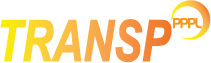z TRANSP# Impurities

## Multiple impurities model

TRANSP can evolve up to eight impurities, which can be defined either as fraction of the electron density or as input UFILES. In the first case, the profile of the impurity species is assumed to be the same as the electron density profile, in the second case the impurity density profile is used directly in TRANSP.

NLZSIM=.T.
to activate the multiple impurity model
XIMPS(j)
to specify the atomic number of all impurities
AIMPS(j)
to specify the atomic weight of all impurities
NLMINSV
TRUE - neutral beam interaction with impurities is computed for each impurity separately and then combined.
FALSE - the neutral beam interaction with impurities is based on a composite impurity density with an averaged charge and atomic weight.

NOTE: TRANSP uses a definition of ion mass as multiple of the proton mass. If the atomic weight of impurities is given in AMU, then it is normalized to A=1.0079.

NCMULTI
Selects how the NCLASS neoclassical analysis deals with multiple impurities - see the NCLASS_neoclassical section
NLABRIDGE
This setting overwrite the default settings for how TORIC deals with impurities. The default is for TRANSP to pass the lowest-Z and the highest-Z impurity to TORIC, which accepts two impurity species. If NLABRIDGE=.TRUE. for any two impurites j1 and j2, these two impurities are used in TORIC.
NOTE: quasineutrality with the unabridgded list of species will be enforced.

### Defining the impurities via UFILES

The impurity density can be given to TRANSP as input UFILES, with prefix and extension PRESIM(j)/EXTSIM(j). In this case, NRISIM(j) needs to be defined for the radial coordinate and NSYSIM(j) needs to be defined for the profile symmetrization.

The UFILES for impurites can be 1D, 2D, or 3D:

1D UFILE - in this case the profile is assumed to be constant in time, with single charge state defined by XZIMPS.

2D UFILE - these are standard UFILEs, with dimensions space and time (in either sequence). The charge state of this element is defined by XZIMPS.

3D UFILE - these UFILES allow to define multiple charge states. The first two coordinates are space and time (in either sequence) and the third coordinate is the charge state.

NOTE:When using the multiple impurity model, the TRANSP output includes all calculated charge states, independently of the format of the input UFILES or whether the option DENSIM is used.

### Defining the impurities in the namelist

The impurity fraction can be defined in the TRDAT namelist using the variable DENSIM and/or DENSIMA. In this case the impurity profile is assumed to have the same shape as the electron density profile and rescaled according to the value of DENSIM and/or DENSIMA, which are updatable in time. DENSIM indicates the fraction on axis, while DENSIMA indicates the fraction at the edge.

DENSIM
If only DENSIM is defined, then the impurity density is rescaled to satisfy nZ/ne=DENSIM on axis.
DENSIMA
If only DENSIMA is defined, then the impurity density is rescaled to satisfy nZ/ne=DENSIMA at the edge.
DENSIM and DENSIMA
If both DENSIM and DENSIMA are defined, then the impurity profile is defined as
nx/ne = DENSIM + f(x)*(DENSIMA-DENSIM)
where f(x) = xXZFA1 [1 + (XZFA2-XZFA1)(x-1)]
and
f(0)=0, f'(0)=0 for XZFA1 > 1
f(1)=1, f'(1)=XZFA2
The default values are: XZFA1=2, XZFA2=0

NOTE: Impurities can be defined in a mixed form, using DENSIM or the PRESIM/EXTSIM UFILES. If UFILES are provided only for some of the impurities, then DENSIM will be used for those impurites for which an UFILE is not provided. If both DENSIM and an UFILE are defined for any given impurity, then the values of DENSIM/DENSIMA are used to rescale the density in the UFILEs, according to the following rules:

PRESIM,EXTSIM
In this case the density profile, as given in the UFILES, is used.
PRESIM,EXTSIM & DENSIM
In this case, the input profiles are rescaled to satisfy nZ/ne=DENSIM on axis.
PRESIM,EXTSIM & DENSIMA
In this case, the input profiles are rescaled to satisfy nZ/ne=DENSIMA at the edge.
PRESIM,EXTSIM,DENSIM,DENSIMA
In this case the input profiles are rescaled linearly from the axis to the edge according to DENSIM and DENSIMA.

nZ here represents the impurity density, summed over all charge states.

If none of the above is defined, then TRANSP will use the default, original, single impurity model (see below).

IMPORTANT:The default value of DENSIM/DENSIMA is -1, which stands for "inactive" and means that the impurity profiles from the UFILEs are not rescaled. While DENSIM/DENSIMA can be updated in time, to ensure that the impurity density profiles evolve without discontinuities, their values are limited to be either negative or positive. For example, if the initial value of DENSIM/DENSIMA is -1, then updates to positive values are not allowed, and viceversa. This means that either the profiles are rescaled during the entire simulation, or they are not.

## Single impurity model

This is the default model for impurties in TRANSP, which assumes a single, fully stripped species.

XZIMP
atomic number of impurity.
AIMP
atomic weight of impurity

Contrary to the case of multiple impurity species, in this case the impurity density cannot be defined as a fraction of the electron density, but it is determined by simultaneous solution of Zeff and quasi-neutrality equations, where Zeff and the electron density are input to the simulation. The output are the impurity density and the average (over hydrogenic species) ion density.

## Coronal equilibrium

The charge states can be redistributed according to the coronal equilibrium, through the NADVSIM(I) namelist variable control, for each impurity element.